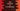# Java program to find the maximum value between two BigInteger### Find maximum value between two BigInteger values using max() method :

In this Java programming tutorial, we will learn how to find the maximum value between two BigInteger values. BigInteger has one inbuilt method max() that will find out the maximum value between two values. The declaration of this method is as below :

``public BigInteger max(BigInteger val)``

The function max() takes one BigInteger as argument and compare it with the other BigInteger and then it will return the maximum value between these two values. Let’s take a look at the below program :

### Java Program :

``````import java.math.BigInteger;

public class Main {

public static void main(String[] args) {
BigInteger firstNumber, secondNumber, maxNumber;

firstNumber = new BigInteger("999");
secondNumber = new BigInteger("111");

maxNumber = firstNumber.max(secondNumber);

System.out.println("The maximum value between " + firstNumber + " and " + secondNumber + " is " + maxNumber);

}

}``````

It will print :

``The maximum value between 999 and 111 is 999``

So, the maxim value between the two bigintegers firstNumber and secondNumber is calculated and store it in the maxNumber variable. Then we have printed it out.